# MP Board Class 11th Maths Important Questions Chapter 2 Relations and Functions

## MP Board Class 11th Maths Important Questions Chapter 2 Relations and Functions

### Relations and Functions Important Questions

Relations and Functions Objective Type Questions

(A) Choose the correct option :

Question 1.
If A = {2, 4, 5}, B = {7, 8, 9}, then n(A × B) =
(a) 6
(b) 9
(c) 3
(d) 0.
(b) 9

Question 2.
If A = { 1, 2, 3, 4, 5} and 5 = {2, 3, 6, 7}, then the number of element in (A × B)∩(B × A) is:
(a) 4
(b) 5
(c) 10
(d) 20.
(a) 4

Question 3.
If A and B are two non – empty sets, then:
(a) A × B = {(a, b) : a ∈ B, b ∈ A}
(b) A × B = {(a, b) : a ∈ A, b ∈ B}
(c) {(a, b) : (a, b) ∈ A, (a, b) ∈ B}
(d) None of these.
(b) A × B = {(a, b) : a ∈ A, b ∈ B}

Question 4.
If f(x) = log $$\frac { 1+x }{ 1-x }$$, then find f [ $$\frac { { 2x } }{ 1+{ x }^{ 2 } }$$ ] =
(a) [f(x)]2
(b) [f(x)]3
(c) 2 f(x)
(d) 3 f(x).
(c) 2 f(x)Question 5.
Let A = {1,2} and B = {3,4}, then the number of relation from A to B will be:
(a) 2
(b) 4
(c) 8
(d) 16
(d) 16

Question 6.
The range of the function f(x) = $$\sqrt { x-1 }$$ is:
(a) [1, ∞)
(b) [0, ∞)
(c) (0, ∞)
(d) (1, ∞)
(b) [0, ∞)

Question 7.
If f(x) = $$\frac { x^{ 2 }-1 }{ x^{ 2 }+1 }$$, then f ( $$\frac{1}{x}$$ ) is:
(a) f(x)
(b) – f(x)
(c) f(-x)
(d) $$\frac { 1 }{ f(x) }$$
(b) – f(x)

Question 8.
Domain of the function f(x) = $$\frac { 1 }{ \sqrt { 2x-3 } }$$ is:
(a) R – { $$\frac{3}{2}$$ }
(b) ( $$\frac{3}{2}$$, ∞)
(c) [ $$\frac{3}{2}$$, ∞)
(d) None of these.
(b) ( $$\frac{3}{2}$$, ∞)

(B) Match the following :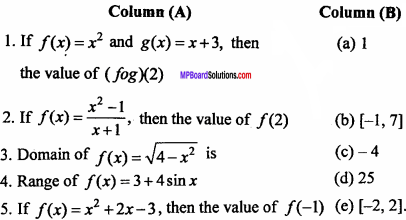1. (d)
2. (a)
3. (c)
4. (b)
5. (c)

(C) Fill in the blanks:

1. If A = {1, 2} and B = {3, 4, 5}, then the number of subsets of A × B is ………………………….
2. Range of the function f = {(2, 1), (3, 1), (4, 1), (5, 1)} is …………………………..
3. Range of the function f(x) = 11 – 7 sinx is …………………………..
4. If f(x) = x2 and g(x) = x + 1, ∀ x ∈ R , then (f + g)x is …………………………
5. If f(x) = 1 – cosx, then the value of f ( $$\frac { \pi }{ 4 }$$ ) …………………………….
6. Domain of the function f(x) = $$\frac { 1 }{ \sqrt { (1 – x)(x – 2) } }$$ is ……………………………
7. If relation R = {(1, 3), (3, 3), (4, 5)} then the value of R-1 is ……………………………

1. 64
2. {1}
3. [4, 18]
4. x2 + x + 1
5. 1 – $$\frac { 1 }{ \sqrt { 2 } }$$
6. (1, 2)
7. {(3, 1), (3, 3), (5, 4)}.

(D) Write true/false :

1. If A, B, C are three sets, then the value of A × (B∪C) is (A∪B) × (A∪C).
2. If A = {x : x2 – 5x + 6 = o}, B = {2,4}, C = {4,5}, then A × (B∩C) = {(2, 4), (3, 4)}.
3. The relation R = {(2, 1), (3, 2), (4, 3), (5, 4)} is a function.
4. If a relation on Z is R = {(x, y) : x, y ∈ Z, x2 + y2 ≤ 4}, then domain of R is {0, ± 1, ± 2}.
5. Domain of the function f(x) = $$\sqrt { a^{ 2 }-x^{ 2 } }$$, a > 0 is [0, a].

1. False
2. True
3. True
4. True
5. False

(E) Write answer in one word/sentence:

1. If f(x) = x2 and g(x) = x + 3, x ∈ R, then the value of (fog)(2).
2. Range of function f(x) = sin x.
3. If mapping f : R → R is defmd by f(x) = x2 + 1 the value of f-1 (26) is:
4. If A = {1, 2, 3} and B = {5, 7}, then the value of A×B is:
5. Domain of the function f(x) = $$\sqrt { 3-2x }$$ is:
6. If function f(x) = $$\frac { { x }^{ 2 } }{ 1-{ x }^{ 2 } }$$, then the value of f(sin θ) is :

1. 25
2. [-1, 1]
3. {-5, 5}
4. {(1, 5), (1, 7), (2, 5), (2, 7), (3, 5), (3, 7)}
5. (-∞, $$\frac { 3 }{ 2 }$$)
6. tan2 θ

Straight Lines Very Short Answer Type Questions

Question 1.
If the set A has 3 elements and the set B = {3, 4, 5}, then find the number of elements in ( A x B). (NCERT)
Solution:
Given : n(A) = 3, B = {3, 4, 5}, n (B) = 3
∴ n (A x B) = n (A) x n (B) = 3 x 3
⇒ n (A x B) = 9.

Question 2.
If G = {7, 8} and H = {5, 4, 2}, then find G x H. (NCERT)
Solution:
G x H = { 7, 8} x {5, 4, 2}
G x H= { (7, 5), (7, 4), (7, 2), (8, 5), (8, 4), (8, 2)}.

Question 3.
If A x B = { (a, x), (a, y), (b, x), (b, y)} then find A and 5. (NCERT)
Solution:
Given:
A x B = {(a, x), (a, y), (6, x), (6, y)}
A = {a, b} and S = {x, y}.Question 4.
If A = {1, 2} and B = {3, 4} then find A x B. (NCERT)
Solution:
A x B = { 1, 2} x {3, 4}
A x B = { ( 1, 3), (1, 4), (2, 3), (2, 4)}

Question 5.
The figure shows the relationship between the set P and Q. Write the relation : (NCERT)

1. In set builder form
2. In roster form
3. Find its domain
4. Find its range.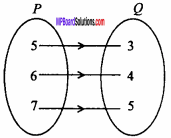Solution:

1. Set builder form, R = {(x, y) : y = x – 2, x ∈ P and y ∈ Q}.
2. Roster form, R = {(5, 3), (6, 4), (7, 5)}
3. Domain = {5, 6, 7}
4. Range = {3, 4, 5}.

Question 6.
Let A = (1, 2, 3, 4, 6} and R be the relation on A defined by
{(a, b) : a, b ∈ A, b is exactly divisible by a } :

1. Write R in roster form,
2. Find the domain of R
3. Find the range of R. (NCERT)

Solution:
Given: A = {1, 2, 3, 4, 6}
1. R = {{a, b): a, be A, b is exactly divisible by a }
R= {(1, 1), (1, 2), (1, 3), (1, 4), (1, 6), (2, 2), (2, 4), (2, 6), (3, 3), (3, 6), (4, 4), (6, 6)}.

2. Domain = {1, 2, 3, 4, 6}.

3. Range = {1, 2, 3, 4, 6}.

Question 7.
Let A = {x, y, z} and B = {1, 2}. Find the number of relations from A to B. (NCERT)
Solution: A = {x, y, z}, B= {1, 2}
n(A) = 3, n(B) = 2
No. of relations from A to B = 2mn = 233/2 = 26
= 64.

Question 8.
A function f is defined by f (x) = 2x – 5, write down the following values : (NCERT)

1. f(0)
2. f(7)
3. f(-3).

Solution:
Given: f(x) = 2x – 5
1. Put x = 0,
f(0) = 2(0) – 5 = 0 – 5 = – 5.

2. Put x = 7,
f(7) = 2 x 7 – 5 = 14 – 5 = 9.

3. Put x = – 3,
f(- 3) = 2(- 3) – 5 = – 6 – 5 = – 11.Question 9.
The function ‘t’ which maps temperature in degree celcius into temperature in degree fehrenheit, it is defined as t(c) = $$\frac { 9c }{ 5 }$$ +32. Find the following : (NCERT)

1. t (0)
2. t (28)
3. t (- 10)
4. Find c, when t (c) = 212.

Solution:
Given :
t(c) = $$\frac { 9c }{ 5 }$$ + 32
1. Put c = 0,
t(0) = $$\frac { 9 × 0 }{ 5 }$$ + 32
⇒ t(0) = 32

2. Put c = 28,
t(28) = $$\frac { 9 × 28 }{ 5 }$$ + 32 = $$\frac { 252}{ 5 }$$ + $$\frac { 32}{ 1 }$$
⇒ t(28) = $$\frac { 252 + 160}{ 5 }$$ = $$\frac { 412}{ 5 }$$

3. Put c = – 10,
t(- 10) = $$\frac { 9 x (- 10)}{ 5 }$$ + 32
⇒ t(- 10) = – 18 + 32 = 14

4. Put t(c) = 212,
212 = $$\frac { 9c }{ 5 }$$ + 32
⇒ $$\frac { 9c }{ 5 }$$ = 212 – 32
⇒ $$\frac { 9c }{ 5 }$$ = 180
⇒ c = $$\frac { 180 x 5 }{ 9 }$$

Question 10.
Find the domain and range of the function f(x) = |x|.
Solution:
f(x) = – |x|, f(x) < 0
Domain of f = R.
Range of f = {y : y ∈ R, y ≤ 0} = (- ∞, 0].

Straight Lines Short Answer Type Questions

Question 1.
Find the domain and range of the function f(x) = $$\sqrt { 9 – { x }^{ 2 } }$$.
Solution:
Given : f(x) = $$\sqrt { 9 – { x }^{ 2 } }$$
Value of f(x) is real, if f(x) ≥ 0
9 – x2 ≥ 0
⇒ – (x2 – 9) ≥ 0
⇒ x2 – 9 ≤ 0
⇒ (x + 3)(x – 3) ≤ 0
∴ Domain = [- 3, 3].

Question 2.
If f(x) = x2, then find $$\frac { f(1.1) – f(1)}{ (1.1 – 1)}$$
Solution:
f(x) = x2
f(1.1) = (1.1)2 = 1.21
f(1) = 12 = 1Question 3.
Find domain of function f(x) = $$\frac { { x }^{ 2 } + 2x + 1 }{ { x }^{ 2 } – 8x + 12 }$$
Solution:
Given function is :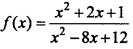f(x) will be defined if,
x2 – 8x + 12 ≠ 0
⇒ x2 – 6x – 2x + 12 ≠ 0
⇒ x(x – 6) – 2(x – 6) ≠ 0
⇒ (x – 2)(x – 6) ≠ 0
x ≠ 2 and x ≠ 6
Domain of function = R – {2, 6}.Question 4.
Let f : g → R → R be defined by f(x) = x + 1 and g(x) = 2x – 3 respectively, then find :

1. f + g
2. $$\frac { f }{ g }$$

Solution:
Given: f(x) = x + 1, g (x) = 2x – 3
1. (f + g)x = f(x) + g(x)
= x + 1 + 2x – 3
∴ (f + g)x = 3x – 2

2. $$\frac { f }{ g }$$(x) = $$\frac { f(x)}{ g(x) }$$ = $$\frac { x +1 }{ 2x – 3}$$

Question 5.
If f(x) = x2 – $$\frac { 1 }{ { x }^{ 2 } }$$, then prove that:
f(x) + f$$\frac { 1 }{ x }$$ = 0
Solution: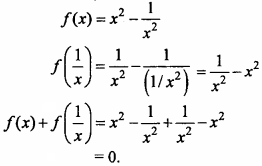Question 6.
If f(x) = $$\frac { { x }^{ 2 } }{ 1-{ x }^{ 2 } }$$, then prove that:
f(sinθ) = tan2 θ.
Solution: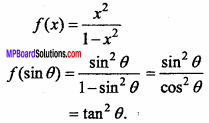Question 7.
If f(x) = x3 + 3x + tanx, then prove that f(x) is an odd function.
Solution:
Given : f(x) = x3 + 3x + tanx
f(- x) = (- x)3 + 3(- x) + tan(- x)
= – x3 – 3x – tanx
= – (x3 + 3x + tanx)
= – f(x).
Hence, f(x) is an odd function.

Question 8.
If f(x) = x2 + 2xsinx + 3, then prove that f(x) is an even function.
Solution:
Given: f(x) = x2 + 2x sin x + 3
f(- x) = (- x)2 + 2(- x) sin(- x) + 3
= x2 + 2xsinx + 3, [∵ sin(- x) = – sinx]
= f(x).
Hence, f(x) is an even function

Question 9.
If f(x) = x2, g(x) = x + 2, ∀ x ∈R, then find gof and fog. Is gof = fog.
Solution:
Given : f(x) = x2, g(x) = x + 2
fog(x) = f[g(x)]
= f(x + 2) = (x+2)2.
gof(x) = g[f(x)]
= g(x2) = x2 + 2
Hence, fog(x) ≠ gof(x).

Question 10.
If f(x) = e2x and g(x) = log $$\sqrt {x}$$, x> 0, then find the value of fog(x) and gof(x).
Solution: